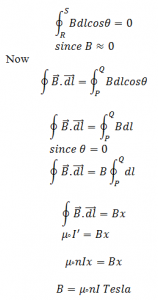# Magnetic Field Due To Solenoid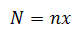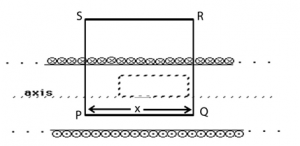Consider a long solenoid having n number of turns per unit length and carrying current I is as shown in figure. The magnetic field outside the solenoid is almost zero but the magnetic field inside the solenoid is uniform which is directed along the axis of the solenoid. Consider a closed loop PQRSP having length PQ= RS = x. If dl be the small line element the direction of B is along dl. So the angle between solenoid and PQ is 0°. If N be the no. of turns in the closed loop PQRSP then,Let I’ be the current enclosed by closed loop then,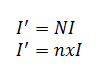Now from Ampere’s Law,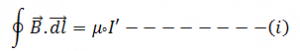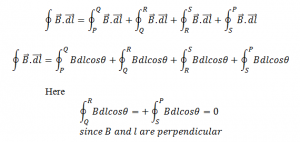and